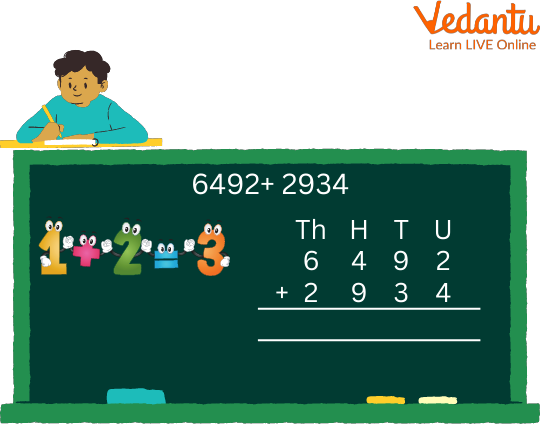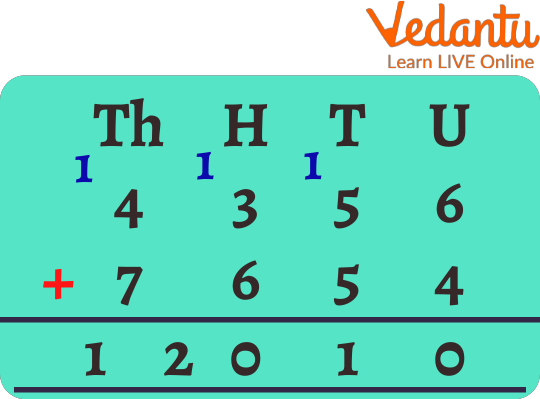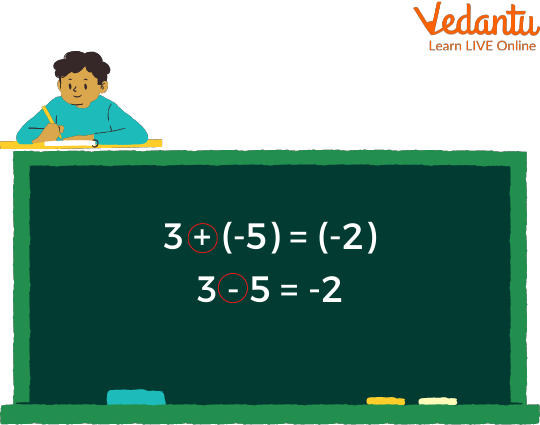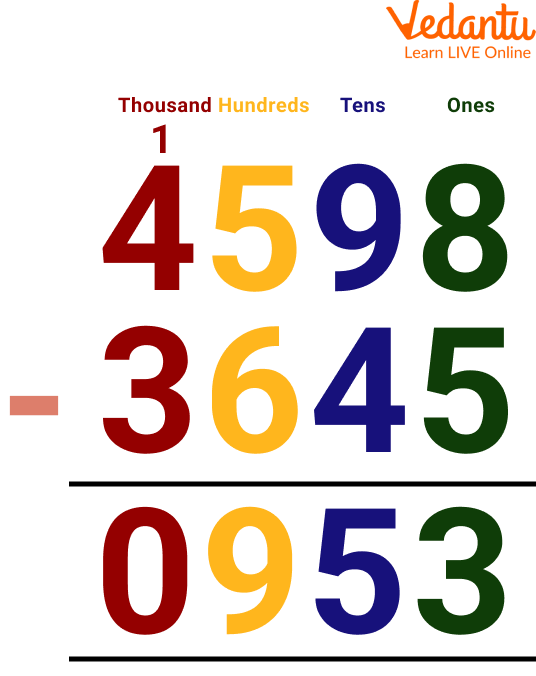Courses
Courses for Kids
Free study material
Free LIVE classes
More

# Addition and Subtraction of 4 digit NumbersLIVE
Join Vedantu’s FREE Mastercalss

## Introduction to Addition and Subtraction of Numbers

In this topic, we are going to learn the concept of addition and subtraction and how we carry out the above process. We will also learn the significance of addition and subtraction in daily life. We will try to conceptualise the addition and subtraction process using the number lines. When we move towards the right in the number line, then addition takes place, and on moving towards the left, subtraction takes place. These are arithmetic operations that we perform in our transactions. In this article, we will also see addition and subtraction of 4-digit numbers worksheets through which you can practice.

## Addition of 4 Digit Number

While adding any number, the basic rule of addition must be followed, which is:

1. Always start adding from the right side, which means the unit digit to the unit digit.

1. If we add two digits and get a two-digit number then the unit digit is written down, and then the tens digit is carried forward and added to the next digits.For example, let us add 4356 and 7654.Example

After adding the unit digits we have got a two-digit number of which the unit digit is written, and the tens digit is carried forward and added to the next digit.

## Subtraction of 4 Digit Number

While subtracting any number from the given number, the basic rule of addition must be followed, which is:

1. We must always subtract a smaller number from a greater number.

1. While subtracting if the digit is smaller than the digit to be subtracted then we take carry over from the next digit and also the subtraction is done from right to left with a unit digit from the unit digit.Subtraction

From the above figure, we can see that, here, the “+(-)” will give the “-” in the result. Therefore, on solving, we get the result as -2.

For example, let us subtract 3456 from 6567.

= 6567 - 3456

= 3111

Here we have subtracted the unit digit from the unit digit, the tens digit from the tens digit, and so on to get the result.

Now let’s see an example of borrowing. Here we can see that 3645 is subtracted from 4598. Here at one's place, 5 is subtracted from 8, and we will get 3. At the tens place, 4 is subtracted from 9, and we will get 5. Similarly, 6 can not be subtracted from 5. Hence, we have to take the borrow 1 from 4 and hundred place 5 will become now 15. So, 15 - 6 = 9

Then 3 - 3 = 0.Subtraction of 4598 - 3645

Basically, addition and subtraction are mathematical operators used to sum up, any two quantities and to find the difference between the two quantities. Imagining a number line, we can say that if we add something, then we move forward in the number line, and while subtracting, we move back on the number line. We can also say that subtraction can be done with the addition process. Suppose we have to find the difference between 34 and 25. One way to find the difference is that subtract 25 from 34, whereas another way is that add and check that on adding what number in 25, we get 34.

## Solved Examples

Q 1. Calculate the total amount Ramesh paid to the shop owner if he bought a pair of trousers worth Rs.4897 and a shirt worth Rs.3450.

Ans: The total amount paid by Ramesh = 4897 + 3450

= 8347

Hence, the total amount paid to the shop owner is Rs.8347.

Q 2. Add the greatest number of 4 digits to the smallest number of 4 digits.

Ans: The greatest number of 4 digits is = 9999

The smallest number of 4 digits is = 1000

The required result of addition is = 9999 + 1000

= 10999

Q 3. If a buyer pays 4 two-thousand rupees notes for an item worth Rs.7234, then what is the amount he will be getting back from the shopkeeper?

Ans: To find the returning amount, we need to subtract the cost from the money given to the shopkeeper.

The money given to the shopkeeper is = 4×2000

= Rs.8000

The cost of the item is = Rs.7234

Returning amount = 8000 - 7234

= 0766

Hence, the shopkeeper will return Rs.766 to the buyer.

## 4 digit Addition and Subtraction Worksheets

Below are some of the 4-digit subtraction worksheets. It also contains 4 digit addition and subtraction word problems worksheets

Q 1. What is the difference between the largest four-digit number and the smallest four-digit number?

Ans: 8999

Q 2. Calculate the total amount paid to the shop owner if a man bought a television worth Rs.9765 and a home theatre worth Rs.3421.

Ans: Rs.13186.

Q 3. Add the difference between 5672 and 4311 to the difference between 7892 and 2134.

Ans: 7119

Q 4. Subtract the sum of 5673 and 5678 with 6783 and 2341.

Ans: 2227

Q 5. Add the largest even 4-digit number to the smallest odd 4-digit number.

Ans: 10999

## Summary

Last updated date: 24th Sep 2023
Total views: 81.6k
Views today: 1.81k

## FAQs on Addition and Subtraction of 4 digit Numbers

1. What will be the result if we add two quantities of the same magnitude but opposite signs?

If we add two quantities with the same magnitude and opposite sign then we will move backward on the number line to the same position from where we have started and we know that we count any number starting from zero so we will get zero.

2. What is the significance of addition in our daily life?

In our day-to-day life we need to buy and sell items for which there is a price and to be efficient in this buying and selling process we need addition and subtraction. Not only this but also we require addition and subtraction everywhere in all aspects of life in experiments, solving any mathematics problem.

3. What is the relation between addition and subtraction?

The addition process is the inverse of the subtraction process. Suppose we add 1 to a number, then we get the successor of the number but on subtracting 1 from the same number we get the predecessor of the number.

But if we add or subtract 0 to any number then the value remains the same.